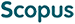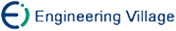熊金志 徐建敏 袁华强. 多项式光滑的支持向量回归机一般模型的收敛性研究[J]. 计算机研究与发展, 2011, 48(3): 464-470.
 引用本文: 熊金志 徐建敏 袁华强. 多项式光滑的支持向量回归机一般模型的收敛性研究[J]. 计算机研究与发展, 2011, 48(3): 464-470.Xiong Jinzhi, Xu Jianmin, and Yuan Huaqiang. Convergenceness of a General Formulation for Polynomial Smooth Support Vector Regressions[J]. Journal of Computer Research and Development, 2011, 48(3): 464-470.
 Citation: Xiong Jinzhi, Xu Jianmin, and Yuan Huaqiang. Convergenceness of a General Formulation for Polynomial Smooth Support Vector Regressions[J]. Journal of Computer Research and Development, 2011, 48(3): 464-470.## Convergenceness of a General Formulation for Polynomial Smooth Support Vector Regressions

• 摘要: 2005年Lee 等人提出光滑的支持向量回归机模型ε-SSVR(smooth ε-support vector regression)，2008年熊金志等人提出一个多项式光滑的支持向量回归机模型ε-PSSVR(polynomial smooth ε-support vector regression),使回归性能及效率得到了一定改善.然而，这种支持向量回归机是否存在一个一般模型，以及一般模型的收敛性等问题没有解决.为此，将一类多项式函数作为新的光滑函数，使用光滑技术，把多项式光滑模型ε-PSSVR推广到一般情形，提出一个多项式光滑的支持向量回归机一般模型ε-dPSSVR(dth-order polynomial smooth ε-support vector regression).并用数学归纳法证明该一般模型的全局收敛性.研究表明：1) 多项式光滑的支持向量回归机存在无穷多个模型，可以用一个一般模型来表示；2) 该一般模型是全局收敛的，其收敛上界比ε-SSVR缩小半个数量级.成功解决了多项式光滑的支持向量回归机的一般形式及其收敛性问题，为进一步研究多项式光滑的支持向量回归机提供了基本的理论支持.

Abstract: Lee et al. proposed a smooth ε-support vector regression (ε-SSVR) in 2005, and Xiong et al. proposed a polynomial smooth ε-support vector regression(ε-PSSVR) in 2008, which improved the performance and efficiency of regression. However, problems still exist in looking for a general formulation of the polynomial smooth ε-support vector regressions and proving its convergenceness. Therefore, using a class of polynomial functions as new smoothing functions, the polynomial smooth model ε-PSSVR is extended to a general case; and a dth-order polynomial smooth ε-support vector regression (ε-dPSSVR), which is a general formulation of polynomial smooth ε-support vector regressions, is proposed using the smoothing technique in this paper. The global convergence of ε-dPSSVR is proved by mathematical inductive method. The research concludes that: 1) there are an infinite number of polynomial smooth models for support vector regression, which can be described in a general formulation; 2) the general formulation is global convergent, and the upper bound of the convergence is reduced about half an order of magnitude to ε-SSVR. The convergence problem of general formulation is successfully solved, which supplies a basic theoretical support for researching polynomial smooth ε-support vector regressions./下载:  全尺寸图片 幻灯片
• 分享
• 用微信扫码二维码

分享至好友和朋友圈